By | September 22, 2020

CBSE Class 11 Physics

NCERT Solutions
Chapter 12
THERMODYNAMICS

1. A geyser heats water flowing at the rate of 3.0 litres per minute from 27 °C to 77 °C. If the geyser operates on a gas burner, what is the rate of consumption of the fuel if its heat of combustion is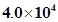J/g?

Ans. Water is flowing at a rate of 3.0 litre/min.

The geyser heats the water, raising the temperature from 27°C to 77°C.

Initial temperature, T1 = 27°C
Final temperature, T2 = 77°C

∴Rise in temperature, ΔT = T2 – T1

= 77°C – 27°C = 50°C

Heat of combustion =4 × 104 J/g

Specific heat of water, c = 4.2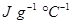Mass of flowing water, m = 3.0 litre/min = 3000 g/min

Total heat used, ΔQ = mc ΔT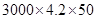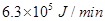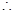Rate of consumption =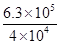= 15.75 g/min

2. What amount of heat must be supplied to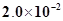kg of nitrogen (at room temperature) to raise its temperature by 45 °C at constant pressure? (Molecular mass of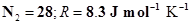.)

Ans. Mass of nitrogen, m = 2.0 × 10–2 kg = 20 g

Rise in temperature, ΔT = 45°C

Molecular mass of N2M = 28

Universal gas constant, R = 8.3 J mol–1 K–1

Number of moles, n = m/M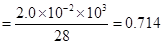Molar specific heat at constant pressure for nitrogen, Cp = (7/2)R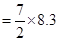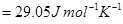The total amount of heat to be supplied is given by the relation: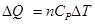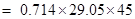= 933.38 J

Therefore, the amount of heat to be supplied is 933.38 J.

3. Explain why

(a) Two bodies at different temperatures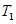and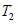if brought in thermal contact do not necessarily settle to the mean temperature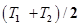.

(b) The coolant in a chemical or a nuclear plant (i.e., the liquid used to prevent the different parts of a plant from getting too hot) should have high specific heat.

(c) Air pressure in a car tyre increases during driving.

(d) The climate of a harbour town is more temperate than that of a town in a desert at the same latitude.

Ans. (a) When two bodies at different temperaturesandare brought in thermal contact, heat flows from the body at the higher temperature to the body at the lower temperature till equilibrium is achieved, i.e., the temperatures of both the bodies become equal. The equilibrium temperature is equal to the mean temperatureonly when the thermal capacities of both the bodies are equal.

(b) The coolant in a chemical or nuclear plant should have a high specific heat. This is because higher the specific heat of the coolant, higher is its heat-absorbing capacity and vice versa. Hence, a liquid having a high specific heat is the best coolant to be used in a nuclear or chemical plant. This would prevent different parts of the plant from getting too hot.

(c) When a car is in motion, the air temperature inside the car increases because of the motion of the air molecules. According to Charles’ law, temperature is directly proportional to pressure. Hence, if the temperature inside a tyre increases, then the air pressure in it will also increase.

(d) A harbour town has a more temperate climate (i.e., without the extremes of heat or cold) than a town located in a desert at the same latitude. This is because the relative humidity in a harbour town is more than it is in a desert town.

4. A cylinder with a movable piston contains 3 moles of hydrogen at standard temperature and pressure. The walls of the cylinder are made of a heat insulator, and the piston is insulated by having a pile of sand on it. By what factor does the pressure of the gas increase if the gas is compressed to half its original volume?

Ans. The cylinder is completely insulated from its surroundings. As a result, no heat is exchanged between the system (cylinder) and its surroundings. Thus, the process is adiabatic.

Initial pressure inside the cylinder =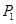Final pressure inside the cylinder =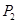Initial volume inside the cylinder =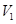Final volume inside the cylinder =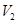Ratio of specific heats,     γ = 1.4

For an adiabatic process, we have: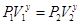The final volume is compressed to half of its initial volume.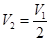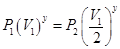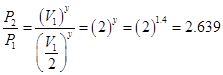Hence, the pressure increases by a factor of 2.639.

5. When changing the state of a gas adiabatically from an equilibrium state A to another equilibrium state B, an amount of work equal to 22.3 J is done on the system. If the gas is taken from state A to B via a process in which the net heat absorbed by the system is 9.35 cal, how much is the net work done by the system in the latter case? (Take 1 cal = 4.19 J)

Ans. The work done (W) on the system while the gas changes from state A to state B is 22.3 J.

This is an adiabatic process. Hence, change in heat is zero.

∴ ΔQ = 0

ΔW = –22.3 J (Since the work is done on the system)

From the first law of thermodynamics, we have:

ΔQ = ΔU + ΔW

Where,

ΔU = Change in the internal energy of the gas

∴ ΔU = ΔQ– ΔW = –(–22.3 J)

ΔU = + 22.3 J

When the gas goes from state A to state B via a process, the net heat absorbed by the system is:

ΔQ = 9.35 cal = 9.35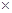4.19 = 39.1765 J

Heat absorbed, ΔQ = ΔU + ΔQ

∴ΔW = ΔQ – ΔU

= 39.1765 – 22.3

= 16.8765 J

Therefore, 16.88 J of work is done by the system.

6. Two cylinders A and B of equal capacity are connected to each other via a stopcock. A contains a gas at standard temperature and pressure. B is completely evacuated. The entire system is thermally insulated. The stopcock is suddenly opened. Answer the following:

(a) What is the final pressure of the gas in A and B?

(b) What is the change in internal energy of the gas?

(c) What is the change in the temperature of the gas?

(d) Do the intermediate states of the system (before settling to the final equilibrium state) lie on its P-V-T surface?

Ans. (a) 0.5 atm

(b) Zero

(c) Zero

(d) No

Explanation:

(a) The volume available to the gas is doubled as soon as the stopcock between cylinders A and B is opened. Since volume is inversely proportional to pressure, the pressure will decrease to one-half of the original value. Since the initial pressure of the gas is 1 atm, the pressure in each cylinder will be 0.5 atm.

(b) The internal energy of the gas can change only when work is done by or on the gas. Since in this case no work is done by or on the gas, the internal energy of the gas will not change.

(c) Since no work is being done by the gas during the expansion of the gas, the temperature of the gas will not change at all.

(d) The given process is a case of free expansion. It is rapid and cannot be controlled. The intermediate states do not satisfy the gas equation and since they are in non-equilibrium states, they do not lie on the PVT surface of the system.

7. A steam engine delivers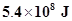of work/minute and services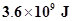of heat/minute from its boiler. What is the efficiency of the engine? How much heat is wasted/minute?

Ans. Work done by the steam engine per minute, W =Heat supplied from the boiler, H =Efficiency of the engine =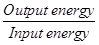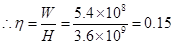Hence, the percentage efficiency of the engine is 15 %.

Amount of heat wasted =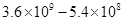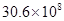Therefore, the amount of heat wasted per minute is.

8. An electric heater supplies heat to a system at a rate of 100W. If system performs work at a rate of 75 Joules per second. At what rate is the internal energy increasing?

Ans. Heat is supplied to the system at a rate of 100 W.

∴Heat supplied, Q = 100 J/s

The system performs at a rate of 75 J/s.

∴Work done, W = 75 J/s

From the first law of thermodynamics, we have:

Q = U + W

Where,

U = Internal energy

U = Q–W

= 100 –75

= 25 J/s

= 25 W

Therefore, the internal energy of the given electric heater increases at a rate of 25 W.

9. A thermodynamic system is taken from an original state to an intermediate state by the linear process shown in Fig. (12.13)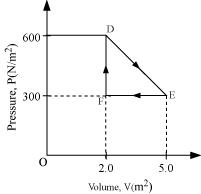Its volume is then reduced to the original value from E to F by an isobaric process. Calculate the total work done by the gas from D to E to F

Ans. Total work done by the gas from D to E to F = Area of ΔDEF

Area of ΔDEF =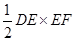Where,

DF = Change in pressure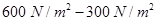= 300 N/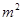FE = Change in volume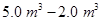= 3.0 m3

Area of ΔDEF = (1/2) × 300 × 3 = 450 J

Therefore, the total work done by the gas from D to E to F is 450 J.

10. A refrigerator is to maintain eatables kept inside at 9°C. If room temperature is 36° C, calculate the coefficient of performance.

Ans. Temperature inside the refrigerator, T1 = 9°C = 282 K
Room temperature, T2 = 36°C = 309 K
Coefficient of performance = T1 / (T2 – T1)
= 282 / (309 – 282)
= 10.44
Therefore, the coefficient of performance of the given refrigerator is 10.44.